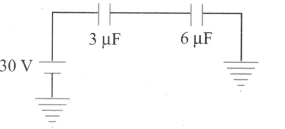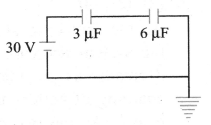Kirchhoff's rules for capacitors
Question

# In the circuit shown in figure, the charge stored on a capacitor of capacitance $3 \mu F$ isModerate
Solution

## Circuit is redrawn as shown in figure. Equivalent capacity of combination is ${C}_{eq}=\frac{3×6}{9}=2 \mu F$Hence, charge$q={C}_{eq}V=2\mu F×30 V=60 \mu C$

Get Instant Solutions